Home MathLab Euler Method Matlab Code

# Euler Method Matlab Code

The Euler method is a numerical method that allows solving differential equations (ordinary differential equations). It is an easy method to use when you have a hard time solving a differential equation and are interested in approximating the behavior of the equation in a certain range.

Here we will see how you can use the Euler method to solve differential equations in Matlab, and look more at the most important shortcomings of the method.

It is to be noted that you can only make use of this method when you have the value of the initial condition of the differential equation you are trying to solve.

Related Post: Free Matlab Alternative

## Euler method

Here are some methods added to the Forward Euler method that falls into the same category while using numerical methods of such: The forward difference, the backward difference, and the central difference method.

### Forward difference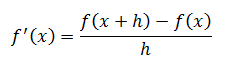### Backward difference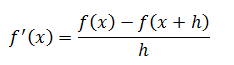### Central difference## Euler Method Matlab

### Forward difference example

Let’s consider the following equation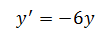The solution of this differential equation is the following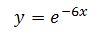What we are trying to do here, is to use the Euler method to solve the equation and plot it alongside with the exact result, to be able to judge the accuracy of the numerical method.

To solve this equation using the Euler method we will do the following

If we rewrite the forward Euler formula above with a different look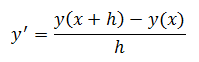Replacing this expression in the equation we are trying to solve will give the following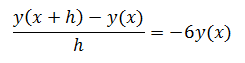If we consider that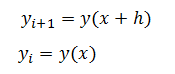And rewrite the equation accordingly, we obtainFeel free to further simplify the expression above, but at this point, we are ready to start coding in Matlab.

#### The Matlab code

```h=0.1; % step's size
N=10; % number of steps
y(1)=1;
for n=1:N
y(n+1)= y(n)+h*(-6*y(n));
x(n+1)=n*h;
end
plot(x,y)```

#### The graph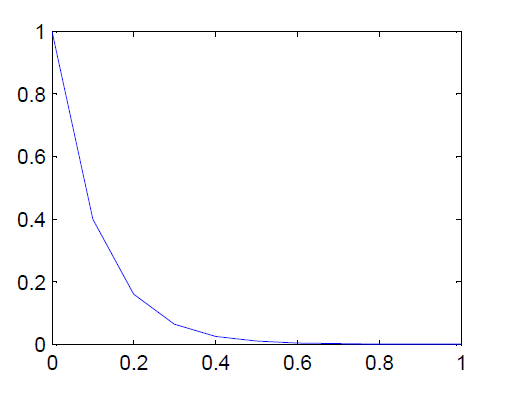Let’s reduce the step’s size and see how it affects accuracy

#### The Matlab  code

```h=0.01; % step's size
N=100; % number of steps
y(1)=1;
for n=1:N
y(n+1)= y(n)+h*(-6*y(n));
x(n+1)=n*h;
end
plot(x,y,'r')```

#### The graph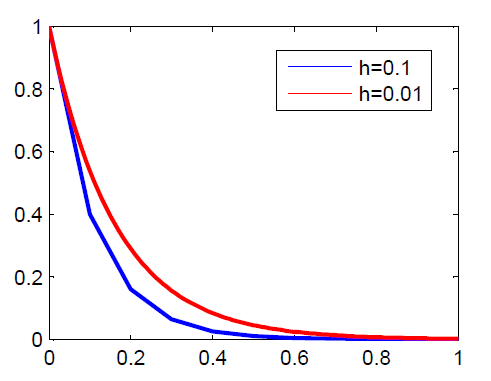This is telling us that when we reduce the value h, it reduces the error. Can we now try comparing our best graph to the exact graph?

Here is the code to help plot the exact graph#### The Matlab code

```x=0:0.001:1;
y=exp(-6.*x);
plot(x,y,'g')```

#### The graphWe can notice by looking at the graph above how both graphs are close to being identical.

For simple functions like the one we just tested, using this Euler method can appear to be accurate especially when you reduce h, but when it comes to complex systems, this may not be the best numerical method to use to approximate the plot of ODEs. Improved methods exist just like the famous Runge-Kutta method.

#### 1 commentOctober 9, 2017 - 3:24 pm

Hi. Could you tell me how to plot two graphs for different step size ?